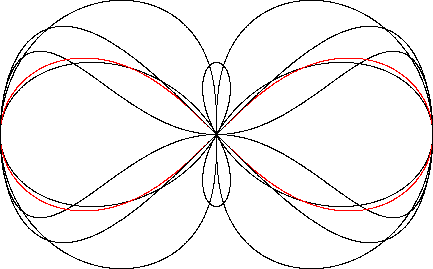Lemniscate of Bernoulli
LEMNISCATE OF BERNOULLI

Balmoral Software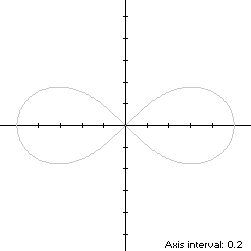The Lemniscate of Bernoulli, or two-leaved rose, is a bisymmetric figure-8 curve S with parametric equationsfor 0 ≤ t < 2π. As t increases from 0, S starts from its right-hand edge at (1,0), moves through Quadrant I to the origin at t = π/2, then clockwise through Quadrant III, its left edge (-1,0) at t = π, and Quadrant II before returning to the origin at t = 3π/2 and passing through Quadrant IV back to its starting point. The ordinate extrema of S are at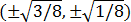when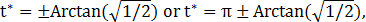so the width x height of its bounding rectangle is### Metrics

The perimeter of S is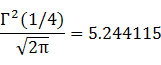and its area is 1.

### Convex Hull

The convex hull is created by connecting the ordinate extrema points with two horizontal line segments of length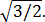We have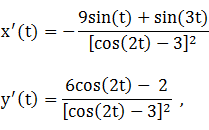so by (L1), the perimeter of the convex hull is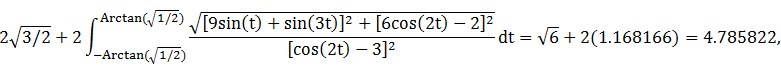which is about 9% shorter than that of the lemniscate.

The line segments of the convex hull create a rectangle of area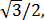as shown in blue in the left diagram below. By (A1), the area of the convex hull is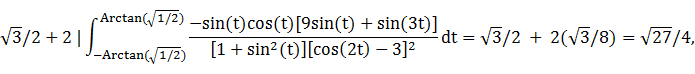which is about 30% more than the area of the lemniscate.

### Circumcircle

The squared-distance function of Sis maximized at 1, so the circumradius is 1.

### Circumellipse

From Lemma B, we have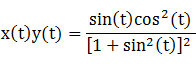This expression is maximized in the first quadrant at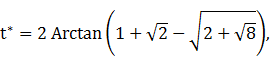so the circumellipse dimensions are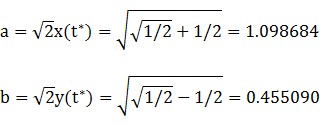For verification, we have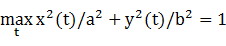### Incircle (lobe)

Consider the right lobe of the lemniscate, where -π/2 ≤ t < π/2. The maximum radius of a circle centered on the x-axis and inscribed in the lobe is the maximum ordinateof S, so a candidate for the lobe incircle has radiusand center abscissaThe candidate circle must be contained within the lobe, so we require that c - R and c + R both be within the abscissa range [0,1] of the lobe, which is true. For verification, we have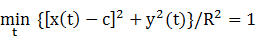### Inellipse (lobe)

Using z = 1 in Lemma E,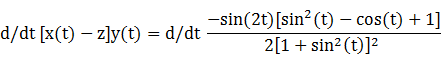has a zero at t* = 0.916671. The corresponding coordinates are
x* = 0.373344

y* = 0.296279

We then have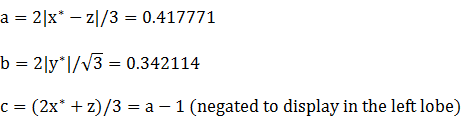For verification,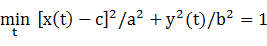### Summary Table

 Perimeter Area Centroid Figure Parameters Incircle (lobe) R =2.221442 0.392699 (0.612372,0)q Inellipse (lobe) a = 0.417771b = 0.342114 2.393169 0.449013 (-0.582229,0) Lemniscate of Bernoulli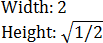5.244115 1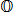Convex hull 4.785822 1.299038 Circumellipse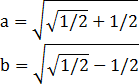5.093049 1.570797 Circumcircle R = 1 6.283185 3.141593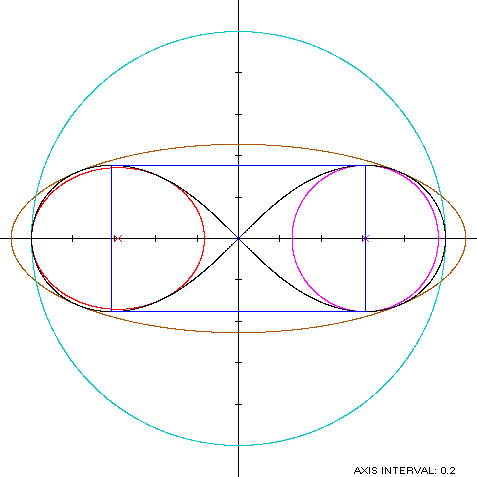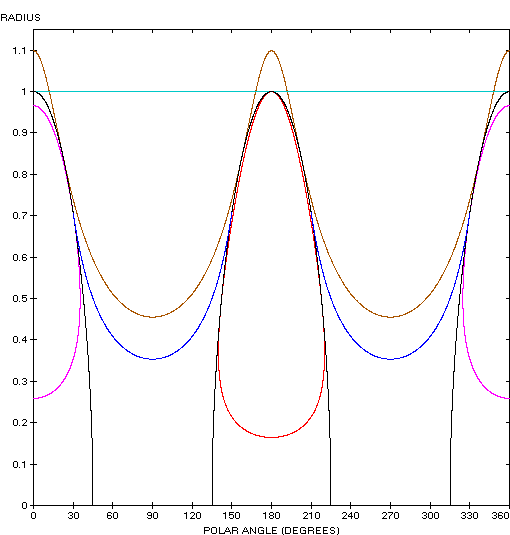Bug-eyed Space Jockey

The Lemniscate of Bernoulli (red) is a member of a group of figure-8 curves described on these pages, including (inside to outside) the dumbbell curve, the bowtie, the Lemniscate of Gerono and the dipole: